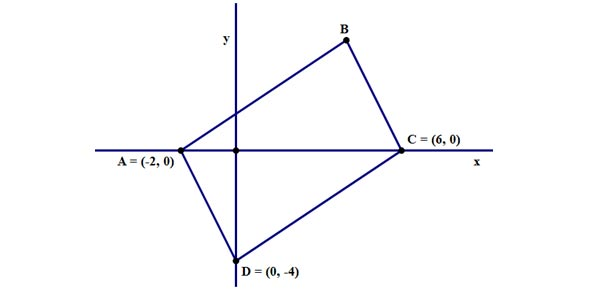# O.M.S. 8th Grade Coordinate Geometry

10 Questions | Total Attempts: 156SettingsCreate your own QuizFind the slope of the line that passes through each pair of points. Use the formula below:

• 1.
(2, 3) (1, 5)
• A.

2

• B.

-2

• C.

1

• D.

-1

• 2.
(-6, 1) (2, 1)
• A.

0

• B.

-0

• C.

Undefined

• D.

-4

• 3.
(3, 0) (5, 0)
• A.

Undefined

• B.

-0

• C.

0

• D.

2

• 4.
(5, 3) (5, -2)
• A.

0

• B.

-1/5

• C.

-5

• D.

Undefined

• 5.
(3, -2) (7, -1)
• A.

-1/4

• B.

1/4

• C.

-4

• D.

4

• 6.
Using the function, y = -5x, what would y equal if x were equal to 0?
• A.

-5

• B.

-1/5

• C.

0

• D.

Undefined

• 7.
Using the function, y = 3x + 1, what would y equal if x were equal to 2?
• A.

5

• B.

-7

• C.

4

• D.

7

• 8.
Using the function, y = -2x + 11, what would y equal if x were equal to 3?
• A.

5

• B.

-5

• C.

17

• D.

-17

• 9.
Using the function, y = 7x - 3, what would y equal if x were equal to 4?
• A.

-5

• B.

25

• C.

31

• D.

-31

• 10.
Using the function, y = -x, what would y equal if x equals -3?
• A.

1

• B.

3

• C.

-3

• D.

-1

Related TopicsBack to top
×

Wait!
Here's an interesting quiz for you.